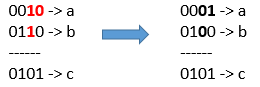# GeetCode Hub

Given 3 positives numbers `a`, `b` and `c`. Return the minimum flips required in some bits of `a` and `b` to make ( `a` OR `b` == `c` ). (bitwise OR operation).
Flip operation consists of change any single bit 1 to 0 or change the bit 0 to 1 in their binary representation.

Example 1:```Input: a = 2, b = 6, c = 5
Output: 3
Explanation: After flips a = 1 , b = 4 , c = 5 such that (`a` OR `b` == `c`)```

Example 2:

```Input: a = 4, b = 2, c = 7
Output: 1
```

Example 3:

```Input: a = 1, b = 2, c = 3
Output: 0
```

Constraints:

• `1 <= a <= 10^9`
• `1 <= b <= 10^9`
• `1 <= c <= 10^9`

class Solution { public int minFlips(int a, int b, int c) { } }